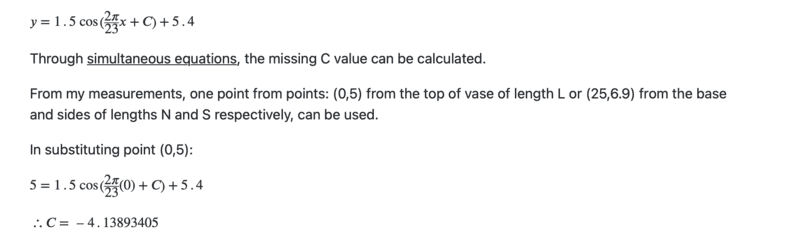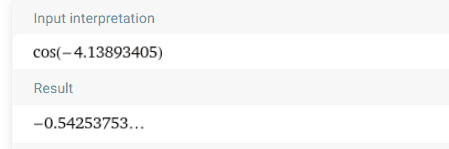# Finding the horizontal shift of a function

• Einstein44
In summary: This means that either the point of the base (25, 4.05) or the top point (0, 5) [must be used to find C]The author has used the point (25, 4.05), not (0, 5) to calculate C.

#### Einstein44

Homework Statement
I was trying to find the horizontal shift of the function, as shown in the picture attached below. It clearly states, that this was found through simultaneous eqn's, but I am unsure how this is done.
Relevant Equations
##y=1.5 cos(\frac{2\pi }{23}x+C)+5.4##I've never actually done this, so I was wondering if someone could show me how this is done. One way I tried was by simply using ##cos^{-1}## in order to cancel the cosine, but that gave me a different value, so I assume this is not how you are supposed to do this.

--> I know I am supposed to know this, but here I am... :)

Yes, he plugged in the x,y values from (0,5) and used ##\cos^{-1}##.
I have no idea what the "From my measurements" line is talking about. It might have something about simultaneous equations, but I can't guess.
I also can't guess why the value of C is that particular solution. There may be some geometry involved that is not shown in your post.

•Einstein44
FactChecker said:
Yes, he plugged in the x,y values from (0,5) and used ##\cos^{-1}##.
I have no idea what the "From my measurements" line is talking about. It might have something about simultaneous equations, but I can't guess.
I also can't guess why the value of C is that particular solution. There may be some geometry involved that is not shown in your post.
Hmmm... This is the website, in case you want to have a look: https://www.ukessays.com/essays/mat...area-of-complex-three-dimensional-objects.php
I can't figure out what kind of geometry could have been used here, as it seems to be a pretty simple scenario to me (and no indication on the website whatsoever), but by calculating this using ##\cos^{-1}## gave me a different value... Have you tried calculating it?

Edit: I believe the "from my measurments" in this case refers to the measurments he took in order to find the rest of the function (a,b,d).

Last edited:
The work on the website is all about the geometry of a vase. That probably determines which of the set of solutions for C are valid in that situation..

•Einstein44
Einstein44 said:
One way I tried was by simply using ##cos^{-1}## in order to cancel the cosine, but that gave me a different value, so I assume this is not how you are supposed to do this.
The closest thing I got to their answer was ##\arccos(-0.4/1.5)-2\pi = 1.8407291226283 - 2\pi = -4.44245618455128##, so I don't know what they did.

•Einstein44
FactChecker said:
The closest thing I got to their answer was ##\arccos(-0.4/1.5)-2\pi = 1.8407291226283 - 2\pi = -4.44245618455128##, so I don't know what they did.

No idea either!?

•Einstein44 and FactChecker
FactChecker said:
The closest thing I got to their answer was ##\arccos(-0.4/1.5)-2\pi = 1.8407291226283 - 2\pi = -4.44245618455128##, so I don't know what they did.
Alright, I guess this will remain a mystery. Thanks for the contribution.

The author has made a mistake. Simple as that!

In the Post #3 link, they say they are using the point (0, 5). But if you look carefully at the diagram
https://images.ukessays.com/180520/3/0651715.022.jpg
the correct point is clearly shown to be (0, 4.586), and this is in fact what has been used to calculate C (not (0,5)).

Easy to confirm: evaluate y using x=0 and the calculated value of C = -4.13893405 and we get:
##y = 1.5 cos(\frac{2\pi }{23}0-4.13893405)+5.4 = 4.5861937##
as required.

••FactChecker and Einstein44
Steve4Physics said:
The author has made a mistake. Simple as that!

In the Post #3 link, they say they are using the point (0, 5). But if you look carefully at the diagram
https://images.ukessays.com/180520/3/0651715.022.jpg
the correct point is clearly shown to be (0, 4.586), and this is in fact what has been used to calculate C (not (0,5)).

Easy to confirm: evaluate y using x=0 and the calculated value of C = -4.13893405 and we get:
##y = 1.5 cos(\frac{2\pi }{23}0-4.13893405)+5.4 = 4.5861937##
as required.
True, that makes sense.

Steve4Physics said:
The author has made a mistake. Simple as that!

In the Post #3 link, they say they are using the point (0, 5). But if you look carefully at the diagram
https://images.ukessays.com/180520/3/0651715.022.jpg
the correct point is clearly shown to be (0, 4.586), and this is in fact what has been used to calculate C (not (0,5)).

Easy to confirm: evaluate y using x=0 and the calculated value of C = -4.13893405 and we get:
##y = 1.5 cos(\frac{2\pi }{23}0-4.13893405)+5.4 = 4.5861937##
as required.
I thought about that too. But the y-ordinate 4.586 is not a measured value. It came from using the (wrongly) calculated sine graph parameters!.

•Steve4Physics
neilparker62 said:
I thought about that too. But the y-ordinate 4.586 is not a measured value. It came from using the (wrongly) calculated sine graph parameters!.
Aha, you are right! I rushed in without reading the text carefully. Apologies to all. But let me try to redeem myself...

In the text of the Post #3 link
https://www.ukessays.com/essays/mat...area-of-complex-three-dimensional-objects.php
the author says:
“This means that either the point of the base (25, 4.05) or the top point (0, 5) [must be used to find C]”.

In fact the author has used the point (25, 4.05), not (0, 5) to calculate C.

##C = cos^{-1}(\frac{(y-5.4)}{1.5}) - \frac{2π}{23}x##

##=cos^{-1}(\frac{(4.05-5.4)}{1.5}) - \frac{2π}{23}25 = -4.1389834##

•Einstein44